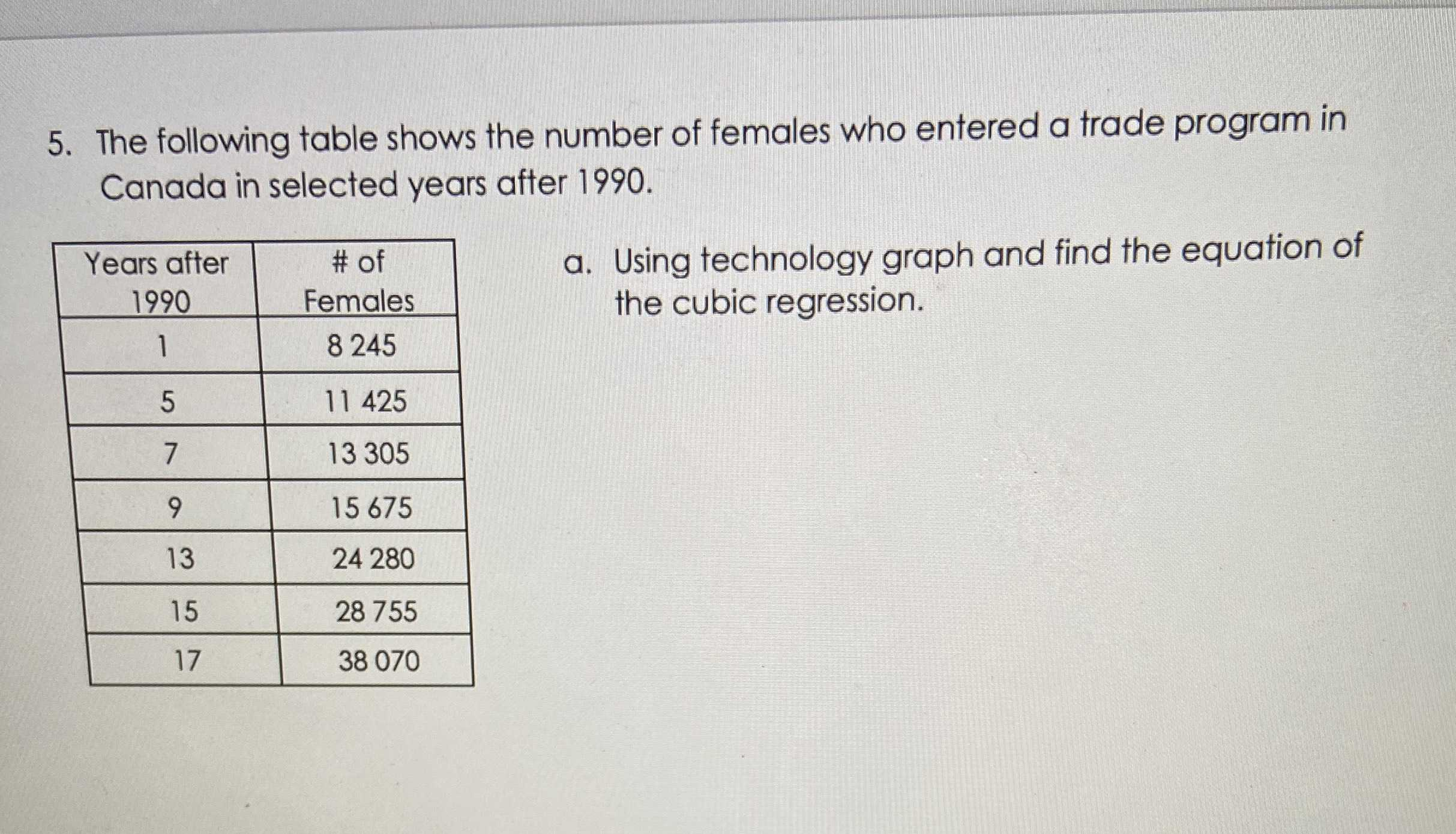### ¿Todavía tienes preguntas de matemáticas?

Pregunte a nuestros tutores expertos
Algebra
Pregunta5. The following table shows the number of females who entered a trade program in Canada in selected years after $$1990 .$$ a. Using technology graph and find the equation of the cubic regression.

$$m = 1.95428 b = - 7.56518$$
$$d = 7181.15$$